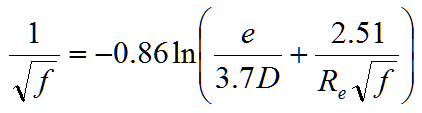hydraulics

# Moody Friction Factor Calculator from Innovyze H2OCalc

3.3 Moody Friction Factor Calculator

Darcy-Weisbach friction factor, f, can be evaluated in terms of equivalent sand grain roughness, e, and Reynolds number, Re. Reynolds number is a dimensionless ratio of inertial forces to viscous forces acting on flow and is defined for any cross-sectional shape asFor Re < 2,000, flow is referred to as laminar; if Re > 4,000, flow is generally turbulent. If Re is between 2,000 and 4,000, the flow is in a transitional region.

For laminar flows, the friction factor, f, is defined asNumerous formulas exist to determine the friction factor. The two most popular equations are the Colebrook-White (implicit) and the Swamee-Jain (explicit). The Colebrook-White equation iswhich must be solved iteratively. Swamee and Jain (1976) developed an explicit formula of the friction factor, f, for 4000 ≤ Re ≤ 108 (turbulent flow region) and 10-6 ≤ e/D ≤ 10-2 asA cubic interpolation from the Moody diagram can be applied for the transitional flow range (2000 ≤ Re ≤ 4000) asProcedure to find friction factor f

First, the relative roughness (e/D) and Reynolds number must be calculated. The Reynolds number is a function of kinematic viscosity of the fluid at the fluid’s temperature. Table 3-2 lists the kinematic viscosity for water over a range of temperature. Then, determine relative roughness of the pipe. Table 3-1 can be used as a guide to estimate equivalent sand-grain roughness for various types of pipes. Then, calculate the friction factor using either of the equations described above depending on the flow regime (i.e. laminar, transitional, or turbulent) based on the Reynolds number.

Table 3-2: Kinematic Viscosity of Water

 Temperature SI (m2/s × 10-7) English (ft2/s × 10-5) T(oC) T(oF) 0 32 17.7 1.91 10 50 13.0 1.40 20 68 10.1 1.09 30 86 8.03 0.86 40 104 6.58 0.71 50 122 5.52 0.59 60 140 4.72 0.51 70 158 4.13 0.44 80 176 3.65 0.39 90 194 3.25 0.35 100 212 2.95 0.32

Source: Boulos et al. (2006)